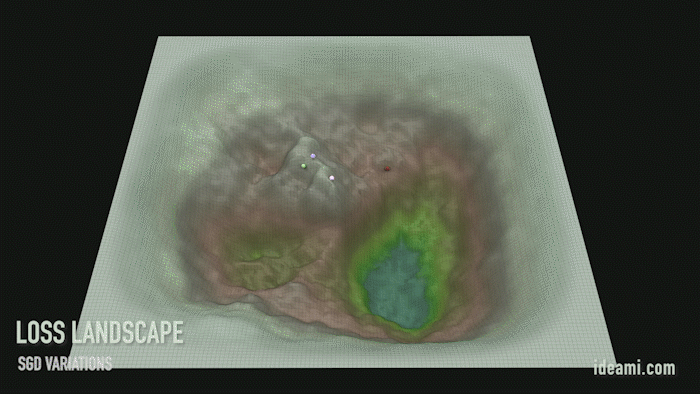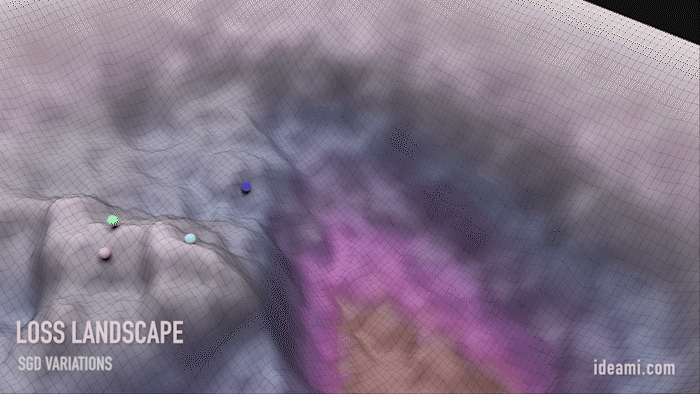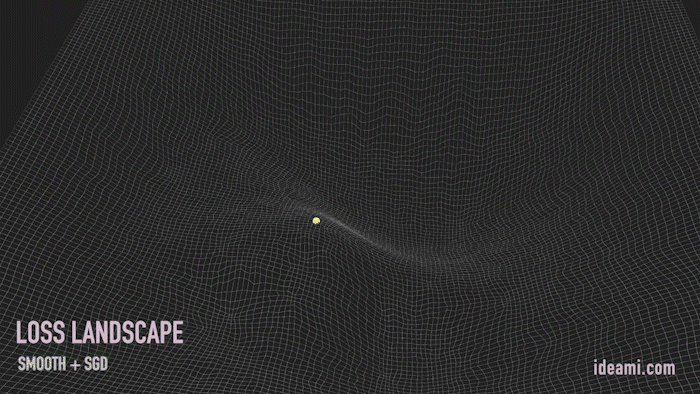# The keys of Deep Learning in 100 lines of code

Source: Deep Learning on Medium

In this 3 part article you are going to:

• Create a neural network from scratch in Python. Train it using the gradient descent algorithm.
• Apply that basic network to The Wisconsin Cancer Data-set. Predict if a tumor is benign or malignant, based on 9 different features.
• Explore deeply how back-propagation and gradient descent work.
• Review the basics and explore advanced concepts. In part 1 we explore the architecture of our network. In part 2, we code it in Python and go deep into back-prop & gradient descent. In part 3, we apply it to the Wisconsin Cancer Data-set.

Let’s go together down the loss landscape of Deep Learning. Ready?Navigating the Loss Landscape within deep learning training processes. Variations include: Std SGD, LR annealing, large LR or SGD+momentum. Loss values modified & scaled to facilitate visual contrast. Visuals by Javier Ideami@ideami.com

These are exciting times for those passionate about the mysteries and possibilities of deep learning.

Many of the heroes in the field share their expertise through videos and articles. They include people like Jeremy Howard at fast.ai, Andrew Ng at Coursera, Andrej Karpathy, Yann Lecun, Ian Goodfellow, Yoshua Bengio, Lex Fridman, Geoffrey Hinton, Jürgen Schmidhuber and many others.

One of the key things that many of them recommend is to get hands on as soon as possible, coding the key principles of deep learning on your own.

Nowadays, we have amazing libraries at our disposal. They include Tensorflow, PyTorch, Fast.ai, Keras, Mxnett, Nctk, DL4J and others. But if you only make use of those powerful libraries, you may be missing out on something important. The chance to reflect deeply about some of the most important parts of these processes.

Coding a net on your own forces you to confront, face to face, the key issues and obstacles in this fascinating adventure. And also, the hidden marvels behind Deep Learning.

Consider all the fancy architectures and latest developments within deep Learning. Convolutions, recurrent networks, GANs and so much more. What is really fascinating is that behind almost every success in the field, we find the same familiar friends. Back-Propagation and Gradient Descent.Navigating the Loss Landscape. Values have been modified and scaled up to facilitate visual contrast.

If you understand deeply those 2 concepts, the sky is the limit. You can then work with the powerful libraries holding a deeper perspective. And you will also be prepared to reflect on your own about new ways in which these tools can be improved.

Ready to begin? It’s going to be quite a ride!Navigating the Loss Landscape. Values have been modified and scaled up to facilitate visual contrast.

### In search of the mystery function

A lot of what happens in the universe can be expressed with functions. A function a mathematical construction that takes an input and produces an output. Cause and effect. Input and Output.

When we look at the world and its challenges, we see information, we see data. And we can learn a lot from that data.

The learning we do with that data can be of different kinds. Let’s highlight 3 very common types within deep learning:

• Supervised learning: When we learn the mystery function from a set of labeled training data. A set of pairs that connect inputs to their corresponding correct outputs.
• Unsupervised learning: When we learn the mystery function from data that is not labeled or classified in any way.
• Reinforcement learning: When we learn that mystery function by maximizing the reward received by an agent. That agent takes actions within an environment.

In this article we will focus on supervised learning, the area where, so far, deep learning has had the most success.

Therefore, we have some data, some input, and we also have some output that corresponds to that input. And we want to understand how inputs and outputs are connected by means of a mystery function.

Thing is, when the data involved reaches a certain complexity, finding that function becomes really hard. Enter neural networks and deep learning.Navigating the Loss Landscape. Values have been modified and scaled up to facilitate visual contrast.

At its heart, a neural network connects your input data and your desired outputs through a series of intermediate “weights”. These weights are really just numbers.

Through their architectures and the optimization algorithms we will soon explore, neural networks become universal approximators. They are able to eventually compute any function that connects their inputs and outputs (when having the right architecture and parameters. To expand on this, see the universal approximation theorem of the mathematical theory of artificial neural networks).

And the best way to understand a neural network is.. to build one! Yes, and from scratch, using the Python programming language in this case. So let’s go for it, and in the process we are going to explore a lot of interesting topics and concepts.

The best way to understand a neural network is.. to build one

Below this paragraph you see the network we will build. It has 2 layers (the input layer is never counted).

• Input: the input of the network contains our source data. The number of neurons matches the number of features of our source data. The graphic below uses 4 input features. We will use 9 when we work later with the Wisconsin cancer data-set.
• First layer: our first hidden layer, it has a number of hidden neurons. Those neurons are connected to all the units in the layers around it.
• Second layer: the second and final layer has 1 single unit, the output of the network.

We could add more layers and have a network with 10 or 20 layers. For simplicity we will work with 2 in this article. A 2 layer neural network can do a lot, as we will find out shortly.

So where will the learning take place within this network?

Let’s recap. In the input layer of our network we put some data. We will also show the network what output corresponds to that input, what result should appear at the output of the network (the second layer).

Each unit within the layers of the network has an associated weight (and a bias, more about that later). Those weights are just numbers that at the beginning of the learning process are typically initialized randomly.

The neural network performs some computations combining the input data with those weights. And those computations spread through the network until they produce a final result at its output.

The result of those computations expresses a function that maps the inputs to the outputs.

What we want is for the network to learn the best possible value of those weights. Because it’s through the computations that the network performs, using those weights in combination with the different layers, that it’s able to approximate different kinds of functions.

Let’s now understand deeper this mystery function that we are looking for. In order to do this, it’s crucial that we clarify with precision the names of all the variables involved in our mission.

• X will represent the input layer, the data we feed to the network.
• Y will represent the target output that corresponds to the input X, the output we should obtain at the end of the network, after it does its computations.
• Yh (y hat) will represent our prediction, the output we produce after we feed X to the network. Therefore, Y is the ideal output, Yh is the output the network produces after we feed it our data.
• W will represent the weights of the layers of the network.

Let’s begin by saying that the first layer, our hidden layer, performs this computation: W X (the product between W and X)

It performs a weighted sum:

• Each unit in a layer is connected to each unit in the previous layer.
• A weight value exists for each of those connections.
• The new value of each unit in a layer becomes the sum of the results of multiplying the value of each previous unit by the weight of the connection between that previous unit and the unit we are currently analyzing.

In a way, the weights express how strong or weak the connections are, the strength of the links between the different units of the network.

And now we are going to add something extra to that product, a bias term:
WX+b

Adding a bias term gives more flexibility to the network. It allows it to “move around” the linear computations of the units, increasing the potential of the network to learn faster those mystery functions.

b: It represents the bias term of the units.

There we have it: WX+ b. This is what we call a linear equation. Linear because it, by means of a product and a sum, represents a linear relationship between the input and the output (a relationship that can be expressed with a line).

Now, remember that a neural network can have multiple layers. In our example we will have 2, but we could have 20 or 200.

Therefore, we will use numbers to indicate to what layer these terms belong. The linear equation that defines the computations of our hidden layer, which is also our layer 1 is: W1 X+ b1

We are going to also give a name to the output of that computation

• Z will represent the output of the computation of a layer

Therefore, Z1 = W1 X+ b1

Notice that this computation should be done for each unit of each layer. When we program the network we will use a vectorized implementation. This means that we will make use of matrices to combine all the computations of a layer within a single mathematical operation.

It’s not essential for this tutorial that you understand matrices in depth, but If you want to refresh your understanding of them, you may check the great videos of 3Blue1Brown and his Essence of Algebra series in YouTube.

So far, so good. Now, imagine a network with many layers. Each of the layers performs a linear computation like the one above. When you chain all those linear computations together, the network is able to compute complex functions.

However, there is a little problem..

### Too linear, too boring

The world is complex, the world is a mess. The relationship between inputs and outputs in real life cannot typically be expressed with a line. It tends to be messy, it tends to be non-linear.

Functions that are complex are often non-linear. And it’s difficult for a neural network to compute non-linear behaviors if it’s architecture is composed of only linear computations. That’s why neural networks add at the end of each of their layers something extra: an activation function.

An activation function is a non-linear function that introduces non-linear changes in the output of the layer. This will ensure that the network is capable of computing all sorts of complex functions, including those that are heavily non-linear.

Now, there are a lot of different kinds of activation functions. Let’s do a quick intro of 4 of the most typical ones.

To explain these activation functions, I need to quickly introduce the concept of the gradient, which we will explore later in depth. The gradient of a function at a point is also called its derivative, and expresses the rate of change of the output of the function at that point.

How much, in what direction and how strongly is the output of the function changing in response to changes in a specific input variable?

When gradients (derivatives) become really small (the output of the function becomes really flat), we talk about vanishing gradients. Later on we will learn that the back-propagation algorithm, heavily used in deep learning, decides how to tweak the values of the weights of the network by using gradients to understand how each parameter of the network is influencing the network’s output (is a change in this parameter making the output of the network increase or decrease?)

Vanishing gradients are a problem because if the gradient at a point becomes too small or zero, it’s very hard to understand the direction in which the output of the system is changing at that point.

We can also talk about the opposite issue, exploding gradients. When the gradient values become very large, the network can become really unstable.

Different activation functions can have different advantages. But they can also suffer of vanishing and exploding gradient issues.

Let’s quickly introduce the most popular activation functions.

Sigmoid
1/(1+e**-x)

• Its output goes from 0 to 1.
• It’s non-linear and it pushes its inputs towards the extremes of its output range. This is great for classifying inputs into two classes, for example.
• Its shape is gentle so its gradient(derivative) will be quite controlled.
• The main disadvantage is that at its extremes the output of the function becomes really flat. This means that its derivative, its rate of change will become really small and the computation efficiency and speed of the units that use this function may slow down or stop altogether.
• Sigmoid, therefore, is useful when present at the final layer of a network because it helps push the output towards 0 or 1 (classifying the output into 2 classes, for example). When used in previous layers, it may suffer of vanishing gradient issues.

Tanh
(2/(1+e**-2x))-1

• Its output goes from -1 to 1.
• It is very similar to Sigmoid, it’s a like a scaled version of it.
• The function is steeper so its derivatives will also be stronger.
• Disadvantages are similar to the ones of the Sigmoid function.

ReLU (rectified linear unit)
max (0,x)

• The output is the input, if the input is above 0. Otherwise, the output is 0.
• Its range goes from 0 to infinity. This means that its output could potentially become very large. There may be issues with exploding gradients.
• A benefit of ReLU is that it can keep the network lighter as some of the neurons may output 0, preventing all the units from being active at the same time (being too dense).
• A problem with ReLU is that its left side is totally flat. This could again produce a gradient, a rate of change, of 0, which can prevent that unit from performing useful computations.
• ReLU computations are simple, cheap to compute.
• Nowadays, ReLUs are the most used activation functions at the inner layers of neural networks.

Softmax
e**x / Sum(e**x)

• The output range is between 0 and 1.
• Softmax normalizes the input into a probability distribution. It compresses the input into a 0 to 1 range like Sigmoid, but it also divides the result so that the sum of all the outputs will be 1.
• It is typically used at the output layer during a multi-classification scenario, when you have to classify the output into multiple classes. Softmax will ensure that the values of the sum of the probabilities associated with each class will always add up to 1.

In this article, we will use the Sigmoid function in our output layer and the ReLU in our hidden layer.

All right, now that we understand activation functions, we need to give them a name!

• A will represent the output of the activation function.

Therefore, at our hidden layer, the computation we perform will be:

A1 = ReLU(Z1)
and Z1=W1 X+b1

And at our second layer, our output layer, the computation will be:

A2 = Sigmoid(Z2)
and Z2=W2 A1 + b2

Notice the use of A1 in the equation of Z2, because the input of the second layer is the output of the first one, which is A1.

Finally, notice that Yh=A2. The output of layer 2 is also the final output of the network.

So that’s it. Now, if we put those computations together, if we chain those functions, we find that the total computation of the neural network is this one:

Yh = A2 = Sigmoid(W2 ReLU (W1 X+ b1) + b2 )

That’s it. That’s the whole computation that our 2 layer neural network performs.

So, in effect, a neural network is a chain of functions, some linear and some non-linear, which together produce a complex function, that mystery function that is going to connect your input data to your desired outputs.

At this stage, notice that out of all the variables in that equation, the values of W and b are the big unknowns. Here is where learning must happen.

Somehow, the network must learn the correct values of W and b that will allow it to compute the correct function.

We will therefore train our network to find the correct values of W1, b1, W2 and b2. But before we can begin that training, we must first initialize those values.

How to initialize the weights and biases of a network is a whole topic in itself and we will go deeper into it later. For now, we are going to initialize them with random values.

At this stage, we can begin to code our neural network. Let’s build a class in Python that initializes its main parameters. Then we will see how we can train it to learn our mystery function.

So let’s jump right into the code in the part 2 of this article and we will be learning and exploring on the go.

Part 2 will be published in 3 days. The link will be added and activated here at that time, see you soon in Part 2 :)